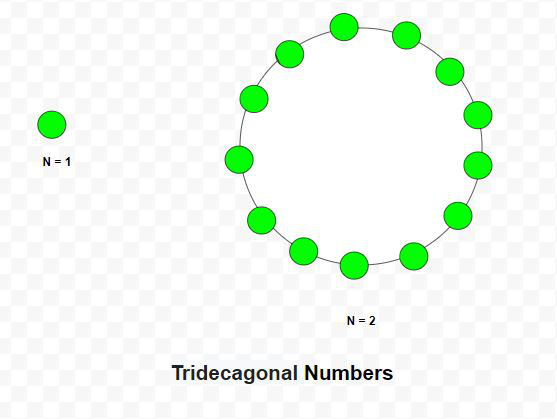# Tridecagonal Number

Given a number N, the task is to find the Nth Tridecagonal number.

A tridecagonal number is a figurate number that extends the concept of triangular and square numbers to the tridecagon(a thirteen-sided polygon). The Nth tridecagonal number counts the number of dots in a pattern of N nested tridecagons, all sharing a common corner, where the ith tridecagon in the pattern has sides made of ‘i’ dots spaced one unit apart from each other. The first few tridecagonal numbers are 1, 13, 36, 70, 115, 171 …

Examples:

Input: N = 2
Output: 13
Explanation:
The second tridecagonal number is 13.

Input: N = 6
Output: 171Approach: The Nth tridecagonal number is given by the formula:

``````

Below is the implementation of the above approach:

## C++

 `// C++ program to find N-th ` `// Tridecagonal number ` ` `  `#include ` `using` `namespace` `std; ` ` `  `// Function to find N-th ` `// Tridecagonal number ` `int` `Tridecagonal_num(``int` `n) ` `{ ` `    ``// Formula to calculate nth ` `    ``// Tridecagonal number ` `    ``return` `(11 * n * n - 9 * n) / 2; ` `} ` ` `  `// Driver Code ` `int` `main() ` `{ ` `    ``int` `n = 3; ` `    ``cout << Tridecagonal_num(n) << endl; ` `     `  `    ``n = 10; ` ` `  `    ``cout << Tridecagonal_num(n) << endl; ` ` `  `    ``return` `0; ` `} `

## Java

 `// Java program to find N-th ` `// tridecagonal number ` `class` `GFG{ ` ` `  `// Function to find N-th ` `// tridecagonal number ` `static` `int` `Tridecagonal_num(``int` `n) ` `{ ` `     `  `    ``// Formula to calculate nth ` `    ``// tridecagonal number ` `    ``return` `(``11` `* n * n - ``9` `* n) / ``2``; ` `} ` ` `  `// Driver Code ` `public` `static` `void` `main(String[] args) ` `{ ` `    ``int` `n = ``3``; ` `    ``System.out.print(Tridecagonal_num(n) + ``"\n"``); ` `     `  `    ``n = ``10``; ` `    ``System.out.print(Tridecagonal_num(n) + ``"\n"``); ` `} ` `} ` ` `  `// This code is contributed by Princi Singh `

## Python3

 `# Python3 program to find N-th  ` `# tridecagonal number  ` ` `  `# Function to find N-th  ` `# tridecagonal number  ` `def` `Tridecagonal_num(n): ` `     `  `    ``# Formula to calculate nth  ` `    ``# tridecagonal number ` `    ``return` `(``11` `*` `n ``*` `n ``-` `9` `*` `n) ``/` `2` ` `  `# Driver Code ` `n ``=` `3` `print``(``int``(Tridecagonal_num(n))) ` ` `  `n ``=` `10` `print``(``int``(Tridecagonal_num(n))) ` ` `  `# This code is contributed by divyeshrabadiya07 `

## C#

 `// C# program to find N-th ` `// tridecagonal number ` `using` `System; ` ` `  `class` `GFG{ ` ` `  `// Function to find N-th ` `// tridecagonal number ` `static` `int` `Tridecagonal_num(``int` `n) ` `{ ` `     `  `    ``// Formula to calculate nth ` `    ``// tridecagonal number ` `    ``return` `(11 * n * n - 9 * n) / 2; ` `} ` ` `  `// Driver Code ` `public` `static` `void` `Main(String[] args) ` `{ ` `    ``int` `n = 3; ` `    ``Console.Write(Tridecagonal_num(n) + ``"\n"``); ` `     `  `    ``n = 10; ` `    ``Console.Write(Tridecagonal_num(n) + ``"\n"``); ` `} ` `} ` ` `  `// This code is contributed by Rajput-Ji `

Output:

```36
505
```

Don’t stop now and take your learning to the next level. Learn all the important concepts of Data Structures and Algorithms with the help of the most trusted course: DSA Self Paced. Become industry ready at a student-friendly price.

My Personal Notes arrow_drop_upCheck out this Author's contributed articles.

If you like GeeksforGeeks and would like to contribute, you can also write an article using contribute.geeksforgeeks.org or mail your article to contribute@geeksforgeeks.org. See your article appearing on the GeeksforGeeks main page and help other Geeks.

Please Improve this article if you find anything incorrect by clicking on the "Improve Article" button below.

Article Tags :
Practice Tags :

Be the First to upvote.

Please write to us at contribute@geeksforgeeks.org to report any issue with the above content.